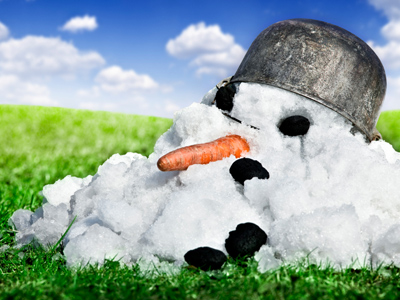The temperature is -13o. If it rises 39o it will be 26o.

# Negative Numbers (Year 6)

In KS2 Maths, you'll learn about negative or minus numbers. In Year Six, it's important to feel comfortable counting up and down in negative numbers and filling in missing numbers in a sequence. You'll also tackle addition and subtraction problems with 2 and 3 figure negative numbers.

Negative numbers are whole numbers below zero. Think about temperatures – if it's colder than 0oC, we say it's a minus temperature. Adding and subtracting with negatives can be tricky, but one trick is to rearrange the problem. For example:

14 - 8 = 6, so 6 - 14 = -8. It gets easier with practice!

Now, have some fun and test your skills with addition and subtraction of negative numbers in this quiz.

1.
The temperature is -15o. If it falls 8o what is the temperature now?
23o
-7o
-23o
7o
15 + 8 = 23 so -15 - 8 = -23
2.
The temperature is -13o. If it rises 39o what is the temperature now?
26o
39o
13o
-26o
39 - 13 = 26 so -13 + 39 = 26
3.
Which of these statements is true?
-33 > -3
-29 > -28
-7 > -17
-44 > -34
The symbol > means greater than
4.
Which is the largest of these numbers?
2
-35
-3
-14
Even the lowest positive number is higher than all the negative numbers
5.
Which of these is the lowest number?
-1
0
-28
-15
Remember that the higher the negative number the less its value
6.
Which are the missing numbers in this sequence? -33, -35, ___, -39, -41, ___?
-36 and -43
-37 and -42
-37 and -43
-37 and -40
The numbers in this sequence are decreasing by 2 each time
7.
The temperature is -28o. If it rises 13o what is the temperature now?
-41o
-15o
15o
41o
28 - 13 = 15 so -28 + 13 = -15
8.
Which of these statements is true?
-1 < -58
-23 < -1
2 < -99
-15 < -20
The symbol < means smaller than
9.
The temperature is 6o. If it falls 18o what is the temperature now?
24o
-24o
-8o
-12o
18 - 6 = 12 so 6 - 18 = -12
10.
Which is the next number in the following sequence? 10, 5, 0, -5
10
-1
-10
-15
The numbers are decreasing by 5